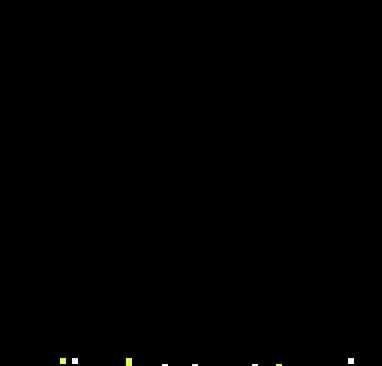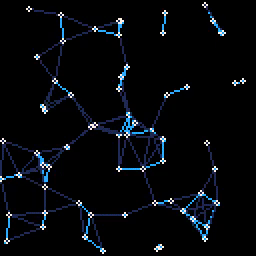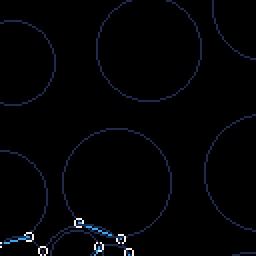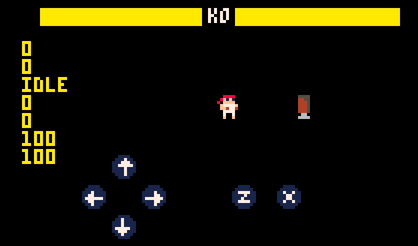# Pico8 demos

pico8, lua

## Introduction

Pico-8 is a fantasy console that let code, create sprites, sfx and music within the same application.

It has some limitations that remind of old school devices and demoscene restrictions which makes developing on it extra fun. For example, the display is 128x128 16 colors, the music has 4 channels, the sprites are 8x8.

Here you can see some of the experiments I have done using it:

## Doom fire

This is based on the article by Fabien Sanglard on how the Doom's fire effect was done.The code was optimized to fit in a tweet:

``poke(0x5F2C,3)f={}p={0,1,2,4,8,9,10,7}w=64d=w*wfunction s(t)e=f[t]i=flr(rnd(3))f[t-i+1-w]=e-band(i,1)endfor i=0,d do f[i]=i>d-w and 8 or 0 endfunction _draw()for x=0,w do for y=0,w-1 do s(y*w+x) end endfor x=0,d do pset(x%w,flr(x/w),p[f[x]]) endend``

You can give it a try at the pico-8 bbs.

## Lines and dots

This one if based on my own implementation of this as you can see in the banner and background of this website.I also optimized its size fit in a tweet as the previous one:

``p={}n=64w=128r=rndfor i=0,n do p[i]={x=r(w),y=r(w),a=r(2)-1,b=r(2)-1}endfunction _draw()cls()for i=0,n doa=p[i]u=a.x v=a.y a.x=(u+a.a)%w a.y=(v+a.b)%wfor j=i,n dob=p[j]x=u-b.x y=v-b.y d=sqrt(x*x+y*y)if(d<20)then line(u,v,b.x,b.y,d<13 and 12or 1)end endcirc(u,v,1,7)endend``

## Circles and connections

This is another simple effect that you can see in the banner and background of this page. I also used it as an example for ECSY.``n=10w=128r=rndfunction p() return (r(w)+(r(2)-1)*t()*10)%w endfunction _draw()	cls()	for i=0,n do	 srand(i)	 l1=r(20)+10	 x=p()	 y=p()	 x=x%w 	 y=y%w	 circ(x,y,l1,1)	 for j=i,n do	 	srand(j)	  l2=r(20)+10		u=p()		v=p()	  dx=u-x	  dy=v-y		d = sqrt(dy*dy+dx*dx)		if d<(l1+l2)and d>abs(l1-l2)then			a=(l1*l1-l2*l2+d*d)/(d*2)			x2=x+dx*a/d			y2=y+dy*a/d			h=sqrt(l1*l1-a*a)			rx=-dy*h/d			ry=dx*h/d			x0=x2+rx			x1=x2-rx			y0=y2+ry			y1=y2-ry			circ(x0,y0,2,7)			circ(x1,y1,2,7)			line(x0,y0,x1,y1,12)		end			 end	endend``

## Pico Fighter

As a huge fan of Street Fighter 2x I started playing around creating a simple base with input to jump, punches and kicks and input buffering to implement special movements like hadokens, shoryukens and tatsumakis.

This is only the early steps, but I really would like to grab some time in the future and expand it and make a playable game.